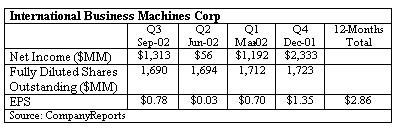### CFA Practice Question

There are 191 practice questions for this study session.

### CFA Practice Question

Justin is trying to estimate the leading P/E multiple of IBM. The current stock price is \$86.20. Justin gathers the following data from the company's financial statements:IBM's current fiscal year ends in December 2002. The expected EPS for the 4th quarter of 2002 and the next fiscal year are \$0.48 and \$3.66, respectively. The leading P/E of IBM is ______.

To calculate the leading P/E multiple, Justin needs the estimated EPS for the next fiscal year. The leading P/E multiple is calculated by dividing the current market price per share by the leading EPS: P/E = Market Price / Leading EPS = \$86.2 / \$3.66 = 23.55

User Comment
prabhur08 If we assume that today is December 31, 2002 then the answer is correct i.e. leading PE is Current Price / Fiscal 2003 earnings. However the question says that the expected EPS for Q4 2002 is \$0.48 So the leading P/E should include Q4 2002 earnings + 3 Quarters of Earnings for 2003 (if today is September 30, 2002) So there is insufficient information to answer the question.
tim2 When you see forward PEs quoted they are usually just the price divided by the estimate for the next year. There's not that much point trying to break it down by quarters as the figures are all just rough estimates that are going to be wrong anyway
tarun2106 thats not really true. If you are in the begining of say Q2, then you would probabily see the next 4 Quarters earnings rather than next Fiscal.
Nando1 I agree with prabhur. One can use either the forecasted earnings for the next 12 months OR the next full-year fiscal period. Therefore, the answer could be 26.73 or 23.55 depending on the method used.
michlam14 i dont even understand what that table is meant for, and for what period it is meant to represent
johntan1979 Table is not needed to calculate leading P/E. All you need is current price of the share and expected EPS for next fiscal year.
gill15 It explicitly says in the notes you can use the NEXT four quarters OR the NEXT fiscal year. We dont have the next four quarters of information directly so choose other.
HolzGe1 good point gill!
CJPerugini Because Q-Q EPS is so volatile, shouldn't we break down EPS growth on a year over year quarterly basis? From that we can forecast 2003Q4 EPS, then determine YOY EPS by (2003 EPS - 2003Q4 EPS + 2002Q4 EPS).
jrojasut09 @gill you hit the nail on the head
walterli This table lmao